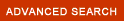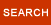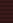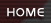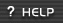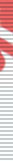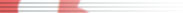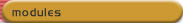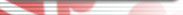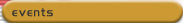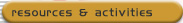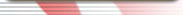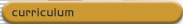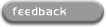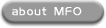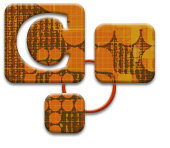Topic C7: Developing Example Test Questions

Introduction
What three decisions must be made before developing an example test question?

What are the types of test questions that measure the ability to recognize an example?

Teaching Suggestions

What three decisions must be made before constructing an example test  question?
Before constructing a test question, an instructor has to make the following three major decisions:

1. Before developing a definition question, it must be decided what will be in the stem of the question: term being defined or the example of the term.

2. What type of question is going to used: multiple choice, true or false, matching, or fill-in-blank.

A multiple choice question should be used for most example questions. Knowledge of any example of a term can be best measured by this type of question.

3. There are two levels of which an example question can be developed. It must be decided at what level the example will be given in the question.

Level one is examples that were given in the textbook or class lecture. Answering a question on this level requires the student memorize the examples given in the class.

Recognizing examples on this level does not measure the student's ability to use the term's identifying characteristics to identify an example of a term.

Questions on this level should be given if the student is expected to recognize something that is already known.

Level two is examples that were not given in the textbook or class discussion. Questions on this level require the student to recognize an example of a term that was not given in the textbook or lecture.

Recognizing an example on this level requires the student to use the term's identifying characteristics to identify the example. Questions on this level require the student to know the term's identifying characteristics.

Questions on this level should be given when students have to recognize  situations or things that can change or more examples can exist than can be given in the textbook or lecture.

What are the types of test questions that measure you ability to recognize an example of a term?
The work done by businesses in our economy places businesses into four different economic groups. Definitions and examples of these four economic groups of businesses will be used to illustrate how to create a test question that measures the ability to recognize an example of a term.

• Extractors are the businesses that take goods from nature. Fishing and farming are examples of extractive businesses.
• Processors are the businesses that change the shape and form of things extracted from nature. Meat packers and paper mills process raw materials into something more useful than they were as a natural resource.
• Assemblers are the businesses that combine the materials made by the processors into some type of product. Airplanes and washing machines are examples of products that were assembled.
• Facilitators are the businesses that repair, store, insure, sell and provide services. Lawn service and banks are examples of businesses that are called facilitators.

The two levels of example questions will be illustrated in the examples of the types of test questions that measure knowledge of examples that follow:

 Objective Test Questions Multiple Choice Question Gives:  Term Response Requires:      Level One - recognize an example given      Level Two - recognize an example not given Level One: Level Two: An example of a business that would be called an extractor is... a.  insurance firm b.  paper mill c.  airplane manufacture d.  fishing answer:  d An example of a business that would be called an extractor is... a.  dental clinic b.  candy manufacture c.  computer repair d.  mining answer:  d

 Question Gives:      Level One - recognize an example given      Level Two - recognize an example not given Response Requires: Recognize term Level One: Level Two: What type of economic activity is fishing? a.  Extractor b.  Processor c.  Assembler d.  Facilitator answer:  a What type of economic activity is mining? a.  Extractor b.  Processor c.  Assembler d.  Facilitator answer:  a

 Question Gives: Term Response Requires: Recognize criteria required to identify something as an example of a term? Level One: Level Two: In order to identify a business as a processor it must... a.  take goods from nature b.  provide services c.  take and shape things taken from nature d.  combine material made by processors into a product. answer:  c This is an important type of test question that is usually never found on a test. As you can see, this question requires the student to know the definition of the term and think how it will be applied to recognize an example.

TOP